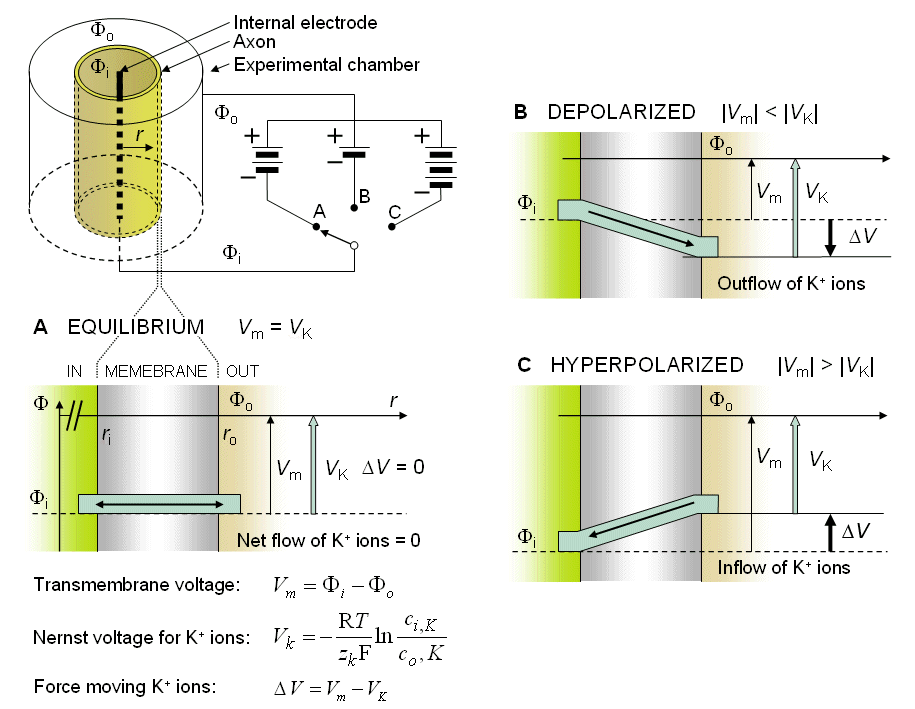Fig. 3.2. An example illustrating the Nernst equation and ion flow through the membrane in(A) equilibrium at rest,(B) depolarized membrane, and(C) hyperpolarized membrane.The diffusional force arising from the concentration gradient is equal and opposite to the equilibrium electric field VK which, in turn, is calculated from the Nernst potential (see Equation 3.21). The Nernst electric field force VK is described by the open arrow. The thin arrow describes the actual electric field Vm across the membrane that is imposed when the battery performs a voltage clamp (see Section 4.2 for the description of voltage clamp). The bold arrow is the net electric field driving force DV in the membrane resulting from the difference between the actual electric field (thin arrow) and the equilibrium electric field (open arrow).